# The stubborn list partition problem

 Importance: Medium ✭✭
 Author(s): Cameron, Kathie Eschen, Elaine M. Hoang, Chinh T. Sritharan, R.
 Subject: Graph Theory » Graph Algorithms
 Keywords: list partition polynomial algorithm
 Prize: none
 Posted by: mdevos on: March 14th, 2007
Problem   Does there exist a polynomial time algorithm which takes as input a graphand for every vertex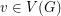a subsetof, and decides if there exists a partition ofinto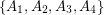so that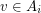only if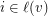and so that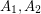are independent,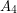is a clique, and there are no edges between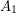and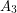?

List partition problems form a very general framework for graph partitioning algorithms. Let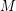be a symmetric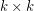matrix over the alphabet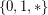. An-partition of a graphis a collection of pairwise disjoint sets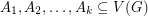with unionwith the following properties. For every, there are no edges between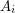andif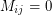, and all possible edges betweenandif. Similarly, for every, the setis independent ifand a clique if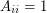. This framework captures a number of interesting problems. For instance, 3-colorability and skew-partitions are represented by the following matrices.So, a graph has an-partition for the first matrix above if and only if it is 3-colorable, and it has a skew partition if and only if it has an-partition for the second matrix above with the added constraint thatare all nonempty.

An even more general framework than-partitions is list-partitions. Here, in addition to amatrix, each vertexof the input graph comes with a list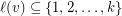of permitted sets. A solution to this problem is an-partition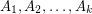with the added property that wheneverwe must have. List-partition problems arise naturally when studying-partitions, since if we decide to put a particular vertexinto a particular set, this will generally place some restrictions on the neighbors and nonneighbors ofwhich can be encoded by lists.

So now, for every possible symmetricmatrix(over) we have a decision problem. Namely, given a graphwith a listfor each vertex, doeshave a list-partition? This problem is quite well understood for matrices of size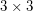and smaller. Here, every such problem is known to either be polynomial solvable, or be NP-complete. In general, Feder and Hell have proven a kind of quasi-dichotomy result for list partition problems. Namely, every such problem is either NP-complete or has a quasi-polynomial time algorithm (whereis the number of vertices, and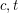are constants depending only on the matrix). Cameron et all investigated the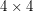matrices and were able to classify all of the associated list partition problems as either polynomial solvable or NP-complete with the exception of two. One is the stubborn problem, specified by the matrix below (and given in the problem statement), the other is the complementary problem (replace's by's and's by's).Hell has mentioned to me (M. DeVos) that he believes this stubborn problem to be the tip of a very interesting iceberg, and he suggested the following problem which is closely related, but perhaps better behaved (it is certainly more symmetric).

Problem: Does there exist a polynomial time algorithm which takes as input a complete graphwith a (not necessarily proper) edge-coloring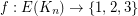and decides if there exists a vertex coloringso that no edge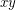has the same color as bothand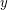?

## Bibliography

[CEHS] K. Cameron, E. Eschen, C.T. Hoàng, R. Sritharan The list partition problem for graphs

* indicates original appearance(s) of problem.

### Solved

http://arxiv.org/abs/1004.5010